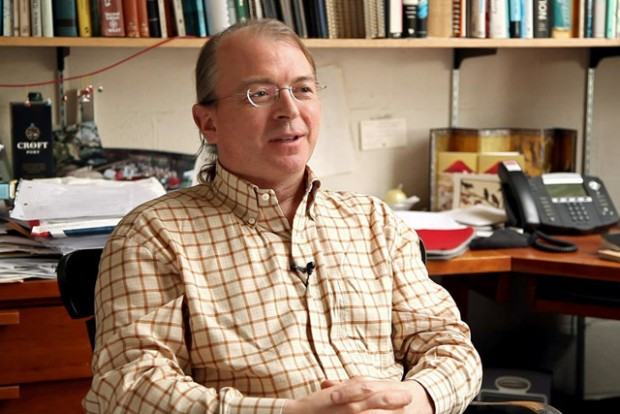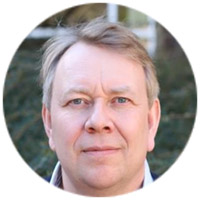Quantum Field Theory and Quantum Topology

Mathematician Jørgen Andersen on topological quantum field theory, path integrals, and quantization of moduli spaces

videos | August 8, 2016

Contemporary Quantum Field Theory is a very active subject. Seen fantastic successes lately, one has a standard model of particle physics which just saw the big success of discovery of the Higgs boson in CERN. In many ways this theory has been validated over and over again. It predicts various physical quantities to the correct ten decimal precision, and so on. There is little doubt that a large part of this theory is just right and it’s doing exactly what it should do in physics.

The problem is that the way that this is described mathematically, or sort of as it were [described] mathematically, is that it uses some infinite dimensional integrals that we do not have a precise mathematical definition for. So quantum field theory is a huge contemporary challenge for mathematics. We need to somehow figure out how to make sense of this.

I would say that we stand in front of – if that’s possible – a big revolution in mathematics, I would think, similar to when Newton and Leibnitz introduced calculus. It must be a completely new machinery which will somehow in many ways revolutionize the way that we do mathematics.Physicist Seth Lloyd on quantum mechanics, classical vs quantum computers and the “universal digital computation”
Let me try to illustrate this by some aspects of quantum topology. So that takes us about thirty years back to when Witten considered a very particular quantum field theory. He took a quantum Chern-Simons theory, and this is a very special quantum field theory, because the theory is such that the Hamiltonian of the theory is zero. So physicists were: «What’s that?», because then there is no dynamics. And that’s right: there is virtually no dynamics. The only dynamics there is, is when the universe goes through a catastrophe when it changes its topology. And so that’s the sort of basic thing about quantum topology is that it is studying quantum field theories which is only senses changes in topology. So Witten proposed that if he took quantum Chern-Simons theory for a certain compact Lie group called su(2). In this theory it is also possible to have observables and these observables are actually not embedded in 3-space and so he was able to device an observable associated to this knot inside 3-space and he was saying: «I can argue with these path integrals that I get an invariant of knots and i don’t just get any invariant, I get the Jones polynomial». And so of course there were lots of people in mathematics who were extremely stunned by this because it was actually an intrinsic 3-dimensional definition of the Jones polynomial as Atiyah had asked for a few years earlier. However, it was using these path integrals that we don’t understand.

But this was quickly after followed by developments by Reshetikhin and Turaev where they used the representation theory of quantum groups to actually rigorously write down all the properties that these quantum field theories should have. This was guided by previous actions by Atiyah, by Segal, and by Witten and they worked out all the actions precisely, they took the representation theory of this quantum group and then they were actually able to show that they could mathematically construct precisely the output of this path integral. And so in that sense we think that these special, very special path integrals are actually being constructed mathematically.

So there was a lot of activity around studying these new quantum field theories which are topological TQFT’s. And it was basically the birth of quantum topology. And one can say that lots of things evolved from here. Many other applications of quantum field theory were made to various parts of mathematics following shortly thereafter.

Several of them had the fantastic feature that one would take a particular mathematical problem that a mathematician had studied and one would turn whatever one had to compute in this theory into some quantum field theory observable or expectation value of some sort. One would then do basic, elementary calculus manipulations to these path integrals, of course non-rigorous, but fine in physics. One would end up with a completely different theory and one would then be able to say that that thing relates to another mathematical precise theory. And one would then simply say: «Well, here are two branches of mathematics that hasn’t connected before, but these two are now connected». And every time we ran into this mathematicians from the two areas of course hit on this and started working on this and so, indeed, yes, these things are related and hard labor followed to prove that what physicists were actually predicting was indeed happening.

So this has sort of been a theme for quantum topology throughout the last thirty years. In various aspects of it we’ve seen many branches be affected, you know, gates theories in low dimensions. Many, many different areas have benefited strongly from this interaction. And what it has made completely clear to a large body of people who study geometry and topology is that we really somehow should come to grips with what is this quantum field theory. Because it seems to be the tool that could allow us to really make much stronger progress.Physicist Max Tegmark on using mathematics to explain the Universe, and how we could develop software to map all mathematical structures
I would say that if we go back to sort of a TQFT and those kinds of aspects, the really wonderful things that have happened there for example, just to somehow illustrate what I was talking about before, was that shortly after the whole thing was introduced, Witten had actually also proposed that if one took a certain moduli space, the moduli space of a flat connections on the surface, then one should be able to apply geometric quantization to this. And this quantization of this moduli space would give a finite-dimensional vector space and this finite-dimensional vector space would have a certain dimension. And, referring back to the work of Verlinde in conformal field theory and connecting everything up, Witten was proposing that there would be this beautiful Verlinde formula that would compute the dimensions of these what I call holomorphic sections of a certain line bundle over these moduli spaces.

And it was a total surprise to people who were working in the quantization of these moduli spaces. Because the formula was clearly an integer, but the expression given for it was very far from an integer. It was evaluations of constants, signs and so on, the rational fractions of pi. For example, it took the genius of Don Zagier to actually verify with nothing else if you just start with this formula and say: «I want to prove it is an integer». So he was able to do this. And subsequently lots of mathematics was developed to actually verify this Verlinde formula. Turned out to be completely right! Just one example of what I was talking about before, that there are many predictions that come from physics and they clearly seem to be right.

This has spurred a lot of activity into actions between people who were studying moduli spaces, and then people who studied quantum topology, and people who were studying other aspects of quantum field theory, other kinds of quantum field theories that have special properties. So these are supersymmetric theories that have certain twists and when you do these certain twists you get a topological theory. And this is still ongoing today. By no means has this been fully explored. We are still discovering that there are lots of very interesting aspects of moduli spaces that are related, so geometric langlands seem to be related to a quantum field theory that Kapustin and Witten have proposed some years ago which are, indeed, sorts of topological twist of Yang-Mills theory. And basically also now we are starting to realize that we can actually also quantize the moduli spaces from non-compact groups using much of the same techniques and we are really starting to try to see if there isn’t also a topological quantum field theory for non-compact gage groups. And these are sort of opening up relations to the Higgs bundle moduli spaces and new Verlinde formulae are actually coming out right now. There is some recent work where we actually have to derive new Verlinde formulae in this non-compact setting which uses a certain equivariant version of the index formula due to Teleman and Woodward to actually prove that these new Verlinde formulae are actually coming out.

But I would say that the big challenge in the field is to really try to at first go take all of these existing quantum field theories and their precise mathematical realizations and understand that all of the tools that are being used in quantum field theory can really be verified.

So, good example is the perturbative expansions that these path integrals are supposed to have. This is the Feynman program for understanding these path integrals, understanding them in terms of sums of graphs. And this is the one that’s only available for the standard model. But that’s not only available in the theories I’m talking about here, because we actually have an exact description of the partition function and of the quantum observables. So we have an option to actually try to understand that the whole thing satisfies these perturbative rules and perturbative expansions summing all the graphs that Feynman was proposing. And this is happening in certain cases and research is going on in this direction.Theoretical physicist Xavier Bekaert on quantum interaction, coupling constant, and properties of gravity
But also another aspect of this which seems to be appearing is that certain quantum field theories seem to have a specific special limit where they in three dimensions seize geometrically very symmetric structures. What I have in mind here is Kashaev’s volume conjecture which says that the asymptotic expansion was a certain quantum invariant actually limits to the hyperbolic volume of a three-manifold. And this hyperbolic volume arises out of the Thurston’s geometrization program which was proved by Perelman which really establishes that all three-manifolds can be decomposed into pieces and each of these pieces has very ideal geometric structures. And slowly it seems to be the case that we are starting to understand that these complicated path integrals are kind of sampling these very beautiful and symmetric geometries in a certain way and they are just somehow someway to repackage this very symmetric information.

And so we’re basically seeing that more and more aspects of quantum field theory seem to be relating back to ideal shapes, to topology and geometry of spaces. And the effort could be really a challenge to try to see if one can turn the picture round and actually understand that all of these averages must have expressions which are much simpler and we simply just are sampling ideal geometry of various configurations. And so of course it would be an ultimate challenge to take these back to the standard model and to see if the similar picture might be amenable there. This I think is out of reach at the moment but I think it’s a challenge and it certainly remains a challenge for mathematics to understand how do we define quantum field theory.Professor of Mathematics, Aarhus University
Did you like it? Share it with your friends!
Published items
0779
To be published soon
+92

Most viewed

New